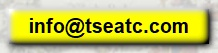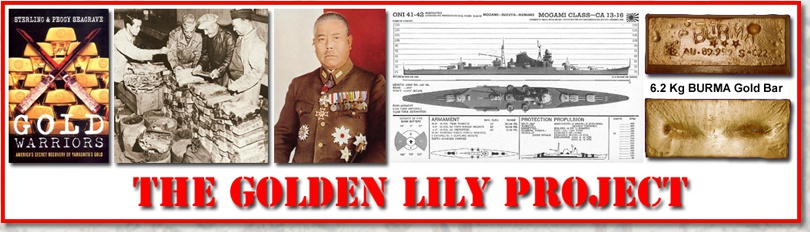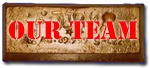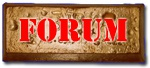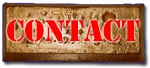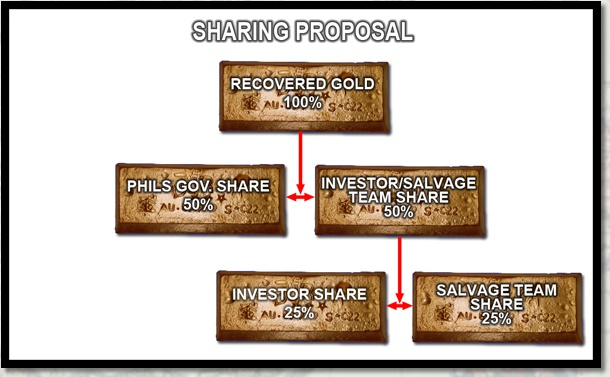INVESTMENT OPPORTUNITIES
In case you’re saying to yourself, “Yes this seems to be a quite exciting project but how will it benefit me if I invest in it?” We will tell you how as follows;

· All investors will be will kept up to date regarding the latest news of this project.

· If and once we make a successful recovery – the investors will get a 50% share back. You may be asking: “50% percent of what?" The sharing will work like this;

1. 50% goes to the Philippine Government.

2. The balance 50% becomes OUR 100% whereby 50% of this will go to the INVESTORS and 50% to the Salvage/Recovery Team.
So, to put it in even simpler terms – Our first target wreck has 200 Metric Tons of Gold on board so if we recover it all then our TOTAL share will be 100 Metric Tons. So, how much is 100 Metric Tons worth? This answer is going to fluctuate daily based on current gold prices so we will go with today’s (March 25, 2015) gold price of USD\$1,198 per ounce.

· One Kilogram = 35.274 Ounces

· \$1,198 x 35.274 = \$42,258.25 Price Per Kilograms

· Once Metric Ton = 1,000 Kilograms so \$42,258.25 x 1,000 = \$42,258,252.00 Price Per Metric Ton (forty two million, two hundred fifty eight thousand, two hundred and fifty two dollars!!!)

· \$42,258,252.00 (Per MT) x 100 (MT) = \$4,225,825,200 Total – 50% of which will go to the Investors (50% = \$2,112,912,600)

OK, so now we know that upon a 200 MT recovery, the Investors share would be Two Billion, One Hundred Twelve Million, Nine Hundred Twelve Thousand, Six Hundred Dollars (\$2,112,912,600). So what does that mean to you as an Investor? OK, let’s just say (as an example) the total project recovery costs Ten Million Dollars (\$10,000,000).

To calculate what each investor would receive we would have to take the total Investors share (\$2,112,912,600) and divide that by the total investment amount (\$10,000,000) and that would be the RETURNS BACK PER EACH DOLLAR to EACH Investor.

\$2,112,912,600 divided by \$10,000,000 = \$211.29 (two hundred eleven dollars and twenty nine cents). So, in this case a \$1,000 investment would get a return back of Two Hundred Eleven Thousand, Two Hundred and Ninety Dollars (\$211,290)! Not a bad return for a \$1,000 Investment!